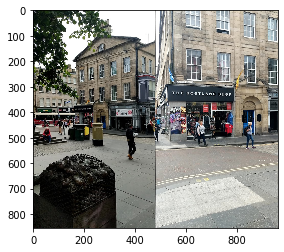## Intro

In the previous tutorial we have created a pipeline for submitting to the IMC2021. However, we have not covered all the possibilities. First, we haven't submissted custom matches, instead we just run a standard Lowe SNN test with cross-check. Second, we haven't evaluate multiview (COLMAP) part. In this tutorial we will do both. I am assuming that you have completed the previous part. If not, please do, because we will be relying on the already extracted features. Let's check if the feature are there

cd imc2021-sample-kornia-submission

angles.h5  descriptors.h5  keypoints.h5  scales.h5  scores.h5

Warning: There is a subtle problem with previous tutorial, so we cannot use pre-extracted features.
Specifically, because OpenCV SIFT does not exactly respect max_features parameter and can sometimes output 8002 features, instead of 8000. When we were importing the features alone, benchmark import_features.py script automatically re-sorted features based on the score and clip the extra 1-3 features. However, this functionality is not available for the importing custom matches. I have already corrected previous post, so you can use it for the re-extration. Or, if you are reading this whole tutorial after May 24, just ignore this.

Now we will install AdaLAM - one of the winners of IMC2020 Challenge. It uses keypoint geometry to filter out unreliable matches.

pip install git+https://github.com/cavalli1234/AdaLAM.git


Let's check if it works on the sample image pair. We will read the pre-extracted features for it.

import matplotlib.pyplot as plt
import numpy as np
import cv2
import os
import torch
import kornia as K
import kornia.feature as KF
import h5py
import json
from PIL import Image
from kornia_moons.feature import *

try:
with h5py.File(filename, 'r') as f:
keys = [key for key in f.keys()]
for key in keys:
except:
print('Cannot find file {}'.format(filename))



I have selected two images, which are matching:

IMG_DIR = '../imc-2021-data/googleurban/edinburgh/set_100/images/'
img1_key = '2b5315968bc5468c995b978620879439'
img2_key = '6264aee21d1b48b7985901c4bedfdbd4'

plt.imshow(np.concatenate([img1, img2], axis=1))

<matplotlib.image.AxesImage at 0x7fa29003f550>We will start with matching and drawing the matches with OpenCV for sanity check.

def opencv_from_imc(kps, sizes, angles):
return [cv2.KeyPoint(kp, kp, float(s), float(a)) for  kp, s, a in zip(kps, sizes, angles)]

def get_data(kps, angles, scales, descs, img_key):
kp1 = kps[img_key]
s1 = scales[img_key]
a1 = angles[img_key]
descs1 = descs[img_key]
return kp1, s1, a1, descs1

def match(img1_key, img2_key, kps, angles, scales, descs):
kp1, s1, a1, descs1 = get_data(kps, angles, scales, descs, img1_key)
kp2, s2, a2, descs2 = get_data(kps, angles, scales, descs, img2_key)
dists, idxs = KF.match_smnn(torch.from_numpy(descs1), torch.from_numpy(descs2), 0.9)
return dists, idxs

def draw_matches(img1_key, img2_key, dists, idxs, kps, angles, scales, descs):
tentatives = cv2_matches_from_kornia(dists, idxs)
draw_params = dict(matchColor = (255,255,0), # draw matches in yellow color
singlePointColor = None,
matchesMask = [True for x in idxs], # draw only inliers
flags = 2)
kp1, s1, a1, _ = get_data(kps, angles, scales, descs, img1_key)
kp2, s2, a2, descs2 = get_data(kps, angles, scales, descs, img2_key)

img_out = cv2.drawMatches(img1,opencv_from_imc(kp1, s1, a1),
img2,opencv_from_imc(kp2, s2, a2),
tentatives,None,**draw_params)
plt.figure()
fig, ax = plt.subplots(figsize=(15, 15))
ax.imshow(img_out, interpolation='nearest')
return

dists, idxs = match(img1_key, img2_key, kps, angles, scales, descs)
draw_matches(img1_key, img2_key, dists, idxs,  kps, angles, scales, descs)

<Figure size 432x288 with 0 Axes>